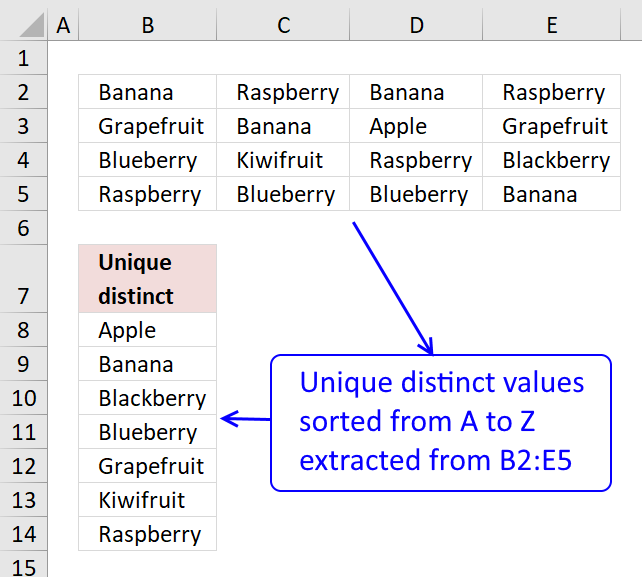Author: Oscar Cronquist Article last updated on November 26, 2018The image above shows an array formula in cell B8 that extracts unique distinct values sorted alphabetically from cell range B2:E5.

Array formula in B8:

=INDEX(\$B\$2:\$E\$5, SMALL(IF(SMALL(IF(COUNTIF(\$B\$7:B7, \$B\$2:\$E\$5)=0, COUNTIF(\$B\$2:\$E\$5, "<"&\$B\$2:\$E\$5)+1, ""), 1)=COUNTIF(\$B\$2:\$E\$5, "<"&\$B\$2:\$E\$5)+1, ROW(\$B\$2:\$E\$5)-MIN(ROW(\$B\$2:\$E\$5))+1), 1), MATCH(MIN(IF(COUNTIF(\$B\$7:B7, \$B\$2:\$E\$5)>0, "", COUNTIF(\$B\$2:\$E\$5, "<"&\$B\$2:\$E\$5)+1)), INDEX(COUNTIF(\$B\$2:\$E\$5, "<"&\$B\$2:\$E\$5)+1, SMALL(IF(SMALL(IF(COUNTIF(\$B\$7:B7, \$B\$2:\$E\$5)=0, COUNTIF(\$B\$2:\$E\$5, "<"&\$B\$2:\$E\$5)+1, ""), 1)=COUNTIF(\$B\$2:\$E\$5, "<"&\$B\$2:\$E\$5)+1, ROW(\$B\$2:\$E\$5)-MIN(ROW(\$B\$2:\$E\$5))+1), 1), , 1), 0), 1)

Copy cell B8 and paste it to cells below as far as necessary.

To enter an array formula, type the formula in a cell then press and hold CTRL + SHIFT simultaneously, now press Enter once. Release all keys.

The formula bar now shows the formula with a beginning and ending curly bracket telling you that you entered the formula successfully. Don't enter the curly brackets yourself.

### Explaining formula in cell B11

This formula consists of two parts, one extracts the row number and the other the column number needed to return the correct value.

INDEX(referencerowcol)

Step 1 to 6 shows how the row number is calculated, step 7 to 11 demonstrates how to calculate the column number.

#### Step 1 - Prevent duplicates

The COUNTIF function counts values based on a condition or criteria, in this case, we take into account previously displayed values in order to prevent duplicates in our output list.

COUNTIF(\$B\$7:B7, \$B\$2:\$E\$5)=0

becomes

COUNTIF("Unique distinct", {"Banana", "Raspberry", "Banana", "Raspberry";"Grapefruit", "Banana", "Apple", "Grapefruit";"Blueberry", "Kiwifruit", "Raspberry", "Blackberry";"Raspberry", "Blueberry", "Blueberry", "Banana"})

becomes

{0,0,0,0;0,0,0,0;0,0,0,0;0,0,0,0}=0

and returns

{TRUE, TRUE, TRUE, TRUE;TRUE, TRUE, TRUE, TRUE;TRUE, TRUE, TRUE, TRUE;TRUE, TRUE, TRUE, TRUE}.

#### Step 2 - Replace TRUE with the corresponding rank order

The following IF function returns the corresponding sort order if the list had been sorted from A to Z based on the logical expression. FALSE returns "" (nothing).

IF(COUNTIF(\$B\$7:B7,\$B\$2:\$E\$5)=0,COUNTIF(\$B\$2:\$E\$5,"<"&\$B\$2:\$E\$5)+1,"")

becomes

IF({TRUE, TRUE, TRUE, TRUE;TRUE, TRUE, TRUE, TRUE;TRUE, TRUE, TRUE, TRUE;TRUE, TRUE, TRUE, TRUE}, {1,12,1,12;9,1,0,9;6,11,12,5;12,6,6,1}+1,"")

becomes

IF({TRUE, TRUE, TRUE, TRUE;TRUE, TRUE, TRUE, TRUE;TRUE, TRUE, TRUE, TRUE;TRUE, TRUE, TRUE, TRUE}, {1,12,1,12;9,1,0,9;6,11,12,5;12,6,6,1}+1,"")

becomes

IF({TRUE, TRUE, TRUE, TRUE;TRUE, TRUE, TRUE, TRUE;TRUE, TRUE, TRUE, TRUE;TRUE, TRUE, TRUE, TRUE}, {2,13,2,13;10,2,1,10;7,12,13,6;13,7,7,2},"")

and returns

{2,13,2,13;10,2,1,10;7,12,13,6;13,7,7,2}

#### Step 3 - Extract smallest value in array

The SMALL function extracts the k-th small number in array, in this case the second argument (k) is 1.

SMALL(IF(COUNTIF(\$B\$7:B7, \$B\$2:\$E\$5)=0, COUNTIF(\$B\$2:\$E\$5, "<"&\$B\$2:\$E\$5)+1, ""), 1)

becomes

SMALL({2,13,2,13;10,2,1,10;7,12,13,6;13,7,7,2}, 1)

and returns 1.

#### Step 4 - Replace TRUE with the corresponding row number

The IF function uses a logical expression to determine which value (argument) to return.

IF(SMALL(IF(COUNTIF(\$B\$7:B7,\$B\$2:\$E\$5)=0,COUNTIF(\$B\$2:\$E\$5,"<"&\$B\$2:\$E\$5)+1,""),1)=COUNTIF(\$B\$2:\$E\$5,"<"&\$B\$2:\$E\$5)+1,ROW(\$B\$2:\$E\$5)-MIN(ROW(\$B\$2:\$E\$5))+1)

becomes

IF(1=COUNTIF(\$B\$2:\$E\$5, "<"&\$B\$2:\$E\$5)+1, ROW(\$B\$2:\$E\$5)-MIN(ROW(\$B\$2:\$E\$5))+1)

becomes

IF(1={2,13,2,13;10,2,1,10;7,12,13,6;13,7,7,2}, ROW(\$B\$2:\$E\$5)-MIN(ROW(\$B\$2:\$E\$5))+1)

becomes

IF({FALSE,FALSE,FALSE,FALSE;FALSE,FALSE,TRUE,FALSE;FALSE,FALSE,FALSE,FALSE;FALSE,FALSE,FALSE,FALSE}, ROW(\$B\$2:\$E\$5)-MIN(ROW(\$B\$2:\$E\$5))+1)

becomes

IF({FALSE,FALSE,FALSE,FALSE;FALSE,FALSE,TRUE,FALSE;FALSE,FALSE,FALSE,FALSE;FALSE,FALSE,FALSE,FALSE}, {1;2;3;4})

and returns

{FALSE,FALSE,FALSE,FALSE;FALSE,FALSE,2,FALSE;FALSE,FALSE,FALSE,FALSE;FALSE,FALSE,FALSE,FALSE}

#### Step 5 - Find smallest value

The SMALL function finds the smallest value in the array ignoring the boolean values

SMALL(IF(SMALL(IF(COUNTIF(\$B\$7:B7,\$B\$2:\$E\$5)=0,COUNTIF(\$B\$2:\$E\$5,"<"&\$B\$2:\$E\$5)+1,""),1)=COUNTIF(\$B\$2:\$E\$5,"<"&\$B\$2:\$E\$5)+1,ROW(\$B\$2:\$E\$5)-MIN(ROW(\$B\$2:\$E\$5))+1),1)

becomes

SMALL({FALSE,FALSE,FALSE,FALSE;FALSE,FALSE,2,FALSE;FALSE,FALSE,FALSE,FALSE;FALSE,FALSE,FALSE,FALSE},1)

and returns 2. This is the row number we need to extract the correct value from cell range B2:E5.

#### Step 6 - Return array

This step uses the INDEX function to return an array from a given row in cell range B2:E5.

INDEX(COUNTIF(\$B\$2:\$E\$5, "<"&\$B\$2:\$E\$5)+1, SMALL(IF(SMALL(IF(COUNTIF(\$B\$7:B7, \$B\$2:\$E\$5)=0, COUNTIF(\$B\$2:\$E\$5, "<"&\$B\$2:\$E\$5)+1, ""), 1)=COUNTIF(\$B\$2:\$E\$5, "<"&\$B\$2:\$E\$5)+1, ROW(\$B\$2:\$E\$5)-MIN(ROW(\$B\$2:\$E\$5))+1), 1), , 1)

becomes

INDEX(COUNTIF(\$B\$2:\$E\$5, "<"&\$B\$2:\$E\$5)+1, 2, , 1)

becomes

INDEX({2,13,2,13;10,2,1,10;7,12,13,6;13,7,7,2}, 2, , 1)

and returns

{10,2,1,10}

#### Step 7 - Match value in array

This step uses the MATCH function to return the correct column number needed to extract the value we need from cell range B2:E5.

MATCH(MIN(IF(COUNTIF(\$B\$7:B7, \$B\$2:\$E\$5)>0, "", COUNTIF(\$B\$2:\$E\$5, "<"&\$B\$2:\$E\$5)+1)), INDEX(COUNTIF(\$B\$2:\$E\$5, "<"&\$B\$2:\$E\$5)+1, SMALL(IF(SMALL(IF(COUNTIF(\$B\$7:B7, \$B\$2:\$E\$5)=0, COUNTIF(\$B\$2:\$E\$5, "<"&\$B\$2:\$E\$5)+1, ""), 1)=COUNTIF(\$B\$2:\$E\$5, "<"&\$B\$2:\$E\$5)+1, ROW(\$B\$2:\$E\$5)-MIN(ROW(\$B\$2:\$E\$5))+1), 1), , 1), 0)

becomes

MATCH(MIN(IF(COUNTIF(\$B\$7:B7, \$B\$2:\$E\$5)>0, "", COUNTIF(\$B\$2:\$E\$5, "<"&\$B\$2:\$E\$5)+1)), {10,2,1,10}, 0)

becomes

MATCH(1, {10,2,1,10}, 0)

and returns 3. This is the column number we need.

#### Step 8 - Return value

INDEX(\$B\$2:\$E\$5, SMALL(IF(SMALL(IF(COUNTIF(\$B\$7:B7, \$B\$2:\$E\$5)=0, COUNTIF(\$B\$2:\$E\$5, "<"&\$B\$2:\$E\$5)+1, ""), 1)=COUNTIF(\$B\$2:\$E\$5, "<"&\$B\$2:\$E\$5)+1, ROW(\$B\$2:\$E\$5)-MIN(ROW(\$B\$2:\$E\$5))+1), 1), MATCH(MIN(IF(COUNTIF(\$B\$7:B7, \$B\$2:\$E\$5)>0, "", COUNTIF(\$B\$2:\$E\$5, "<"&\$B\$2:\$E\$5)+1)), INDEX(COUNTIF(\$B\$2:\$E\$5, "<"&\$B\$2:\$E\$5)+1, SMALL(IF(SMALL(IF(COUNTIF(\$B\$7:B7, \$B\$2:\$E\$5)=0, COUNTIF(\$B\$2:\$E\$5, "<"&\$B\$2:\$E\$5)+1, ""), 1)=COUNTIF(\$B\$2:\$E\$5, "<"&\$B\$2:\$E\$5)+1, ROW(\$B\$2:\$E\$5)-MIN(ROW(\$B\$2:\$E\$5))+1), 1), , 1), 0), 1)

becomes

=INDEX(\$B\$2:\$E\$5, 3, 2)

and returns "Apple" in cell B8.

### Get Excel *.xlsx file

Extract a unique distinct list sorted alphabetically from a range.xlsx